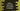# How to find the power of a number using loop in Python## How to find the power of a number using loop in Python:

In this post, we will learn how to find the power of a number using a loop in python. In a production application, you can always use the pow() function to find the power of a number. But if you don’t want to use it, we can create one function that will repeatedly multiply the number to find the power value.

### Find the power by using a for loop:

Let’s try it with a for loop first. Below is the complete program that uses a for loop to find the power:

``````num = int(input("Enter the number: "))
p = int(input("Enter the power: "))

result = 1
for i in range(p):
result *= num

print("Result : {}".format(result))``````

Here,

• num is the number. We are reading its value as an user input.
• p is the value of the power. We are also reading its value as input from the user.
• result is used to store the final result. It is initialized as 1.
• The for loop is used to calculate the final result. It multiplies the value of result with num and assigns that value to result.
• Once the for loop ends, we are printing the value of result.

If you run this program, it will print output as like below:

``````Enter the number: 3
Enter the power: 4
Result : 81``````

## Find the power by using a while loop:

We can also use a while loop to find the power of a number. It works similar to the for loop example we did above. Below is the complete program that uses a while loop to find the power of a number:

``````num = int(input("Enter the number: "))
p = int(input("Enter the power: "))

result = 1
while p > 0:
result *= num
p = p - 1

print("Result : {}".format(result))``````

You can see that it solves the issue similarly. The only difference is that the while loop runs until the value of p is greater than 1. We are decrementing the value of p inside the loop on each iteration. So, it will accumulate the power value at the end of the loop.

If you run it, it will print a similar output.